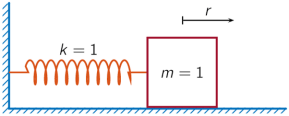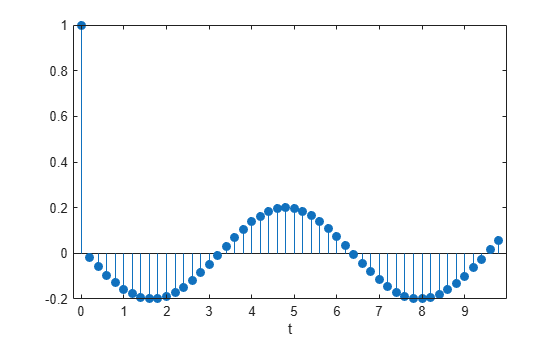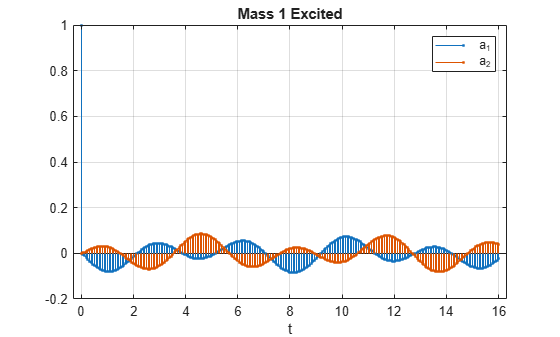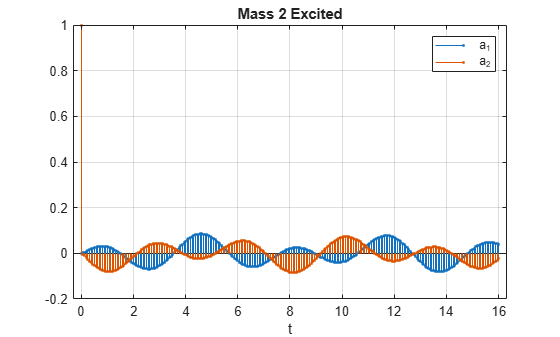Documentation

This is machine translation

Mouseover text to see original. Click the button below to return to the English version of the page.

ss2tf

Convert state-space representation to transfer function

Syntax

``````[b,a] = ss2tf(A,B,C,D)``````
``````[b,a] = ss2tf(A,B,C,D,ni)``````

Description

example

``````[b,a] = ss2tf(A,B,C,D)``` converts a state-space representation of a system into an equivalent transfer function. `ss2tf` returns the Laplace-transform transfer function for continuous-time systems and the Z-transform transfer function for discrete-time systems.```

example

``````[b,a] = ss2tf(A,B,C,D,ni)``` returns the transfer function that results when the `ni`th input of a system with multiple inputs is excited by a unit impulse.```

Examples

collapse all

A one-dimensional discrete-time oscillating system consists of a unit mass, $m$, attached to a wall by a spring of unit elastic constant. A sensor samples the acceleration, $a$, of the mass at ${F}_{s}=5$ Hz.Generate 50 time samples. Define the sampling interval $\Delta t=1/{F}_{s}$.

```Fs = 5; dt = 1/Fs; N = 50; t = dt*(0:N-1);```

The oscillator can be described by the state-space equations

`$\begin{array}{c}x\left(k+1\right)=Ax\left(k\right)+Bu\left(k\right),\\ y\left(k\right)=Cx\left(k\right)+Du\left(k\right),\end{array}$`

where $x={\left(\begin{array}{cc}r& v\end{array}\right)}^{T}$ is the state vector, $r$ and $v$ are respectively the position and velocity of the mass, and the matrices

`$A=\left(\begin{array}{cc}\mathrm{cos}\Delta t& \mathrm{sin}\Delta t\\ -\mathrm{sin}\Delta t& \mathrm{cos}\Delta t\end{array}\right),\phantom{\rule{1em}{0ex}}B=\left(\begin{array}{c}1-\mathrm{cos}\Delta t\\ \mathrm{sin}\Delta t\end{array}\right),\phantom{\rule{1em}{0ex}}C=\left(\begin{array}{cc}-1& 0\end{array}\right),\phantom{\rule{1em}{0ex}}D=\left(\begin{array}{c}1\end{array}\right).$`

```A = [cos(dt) sin(dt);-sin(dt) cos(dt)]; B = [1-cos(dt);sin(dt)]; C = [-1 0]; D = 1;```

The system is excited with a unit impulse in the positive direction. Use the state-space model to compute the time evolution of the system starting from an all-zero initial state.

```u = [1 zeros(1,N-1)]; x = [0;0]; for k = 1:N y(k) = C*x + D*u(k); x = A*x + B*u(k); end```

Plot the acceleration of the mass as a function of time.

```stem(t,y,'filled') xlabel('t')```Compute the time-dependent acceleration using the transfer function H(z) to filter the input. Plot the result.

```[b,a] = ss2tf(A,B,C,D); yt = filter(b,a,u); stem(t,yt,'filled') xlabel('t')```The transfer function of the system has an analytic expression:

`$H\left(z\right)=\frac{1-{z}^{-1}\left(1+\mathrm{cos}\Delta t\right)+{z}^{-2}\mathrm{cos}\Delta t}{1-2{z}^{-1}\mathrm{cos}\Delta t+{z}^{-2}}.$`

Use the expression to filter the input. Plot the response.

```bf = [1 -(1+cos(dt)) cos(dt)]; af = [1 -2*cos(dt) 1]; yf = filter(bf,af,u); stem(t,yf,'filled') xlabel('t')```The result is the same in all three cases.

An ideal one-dimensional oscillating system consists of two unit masses, ${m}_{1}$ and ${m}_{2}$, confined between two walls. Each mass is attached to the nearest wall by a spring of unit elastic constant. Another such spring connects the two masses. Sensors sample ${a}_{1}$ and ${a}_{2}$, the accelerations of the masses, at ${F}_{s}=16$ Hz.Specify a total measurement time of 16 s. Define the sampling interval $\Delta t=1/{F}_{s}$.

```Fs = 16; dt = 1/Fs; N = 257; t = dt*(0:N-1);```

The system can be described by the state-space model

`$\begin{array}{c}x\left(n+1\right)=Ax\left(n\right)+Bu\left(n\right),\\ y\left(n\right)=Cx\left(n\right)+Du\left(n\right),\end{array}$`

where $x={\left(\begin{array}{cccc}{r}_{1}& {v}_{1}& {r}_{2}& {v}_{2}\end{array}\right)}^{T}$ is the state vector and ${r}_{i}$ and ${v}_{i}$ are respectively the location and the velocity of the $i$-th mass. The input vector $u={\left(\begin{array}{cc}{u}_{1}& {u}_{2}\end{array}\right)}^{T}$ and the output vector $y={\left(\begin{array}{cc}{a}_{1}& {a}_{2}\end{array}\right)}^{T}$. The state-space matrices are

`$A=\mathrm{exp}\left({A}_{c}\Delta t\right),\phantom{\rule{1em}{0ex}}B={A}_{c}^{-1}\left(A-I\right){B}_{c},\phantom{\rule{1em}{0ex}}C=\left(\begin{array}{cccc}-2& 0& 1& 0\\ 1& 0& -2& 0\end{array}\right),\phantom{\rule{1em}{0ex}}D=I,$`

the continuous-time state-space matrices are

`${A}_{c}=\left(\begin{array}{cccc}0& 1& 0& 0\\ -2& 0& 1& 0\\ 0& 0& 0& 1\\ 1& 0& -2& 0\end{array}\right),\phantom{\rule{1em}{0ex}}{B}_{c}=\left(\begin{array}{cc}0& 0\\ 1& 0\\ 0& 0\\ 0& 1\end{array}\right),$`

and $I$ denotes an identity matrix of the appropriate size.

```Ac = [0 1 0 0;-2 0 1 0;0 0 0 1;1 0 -2 0]; A = expm(Ac*dt); Bc = [0 0;1 0;0 0;0 1]; B = Ac\(A-eye(4))*Bc; C = [-2 0 1 0;1 0 -2 0]; D = eye(2);```

The first mass, ${m}_{1}$, receives a unit impulse in the positive direction.

```ux = [1 zeros(1,N-1)]; u0 = zeros(1,N); u = [ux;u0];```

Use the model to compute the time evolution of the system starting from an all-zero initial state.

```x = [0;0;0;0]; for k = 1:N y(:,k) = C*x + D*u(:,k); x = A*x + B*u(:,k); end```

Plot the accelerations of the two masses as functions of time.

```stem(t,y','.') xlabel('t') legend('a_1','a_2') title('Mass 1 Excited') grid```Convert the system to its transfer function representation. Find the response of the system to a positive unit impulse excitation on the first mass.

```[b1,a1] = ss2tf(A,B,C,D,1); y1u1 = filter(b1(1,:),a1,ux); y1u2 = filter(b1(2,:),a1,ux);```

Plot the result. The transfer function gives the same response as the state-space model.

```stem(t,[y1u1;y1u2]','.') xlabel('t') legend('a_1','a_2') title('Mass 1 Excited') grid```The system is reset to its initial configuration. Now the other mass, ${m}_{2}$, receives a unit impulse in the positive direction. Compute the time evolution of the system.

```u = [u0;ux]; x = [0;0;0;0]; for k = 1:N y(:,k) = C*x + D*u(:,k); x = A*x + B*u(:,k); end```

Plot the accelerations. The responses of the individual masses are switched.

```stem(t,y','.') xlabel('t') legend('a_1','a_2') title('Mass 2 Excited') grid```Find the response of the system to a positive unit impulse excitation on the second mass.

```[b2,a2] = ss2tf(A,B,C,D,2); y2u1 = filter(b2(1,:),a2,ux); y2u2 = filter(b2(2,:),a2,ux);```

Plot the result. The transfer function gives the same response as the state-space model.

```stem(t,[y2u1;y2u2]','.') xlabel('t') legend('a_1','a_2') title('Mass 2 Excited') grid```Input Arguments

collapse all

State matrix, specified as a matrix. If the system has p inputs and q outputs and is described by n state variables, then `A` is n-by-n.

Data Types: `single` | `double`

Input-to-state matrix, specified as a matrix. If the system has p inputs and q outputs and is described by n state variables, then `B` is n-by-p.

Data Types: `single` | `double`

State-to-output matrix, specified as a matrix. If the system has p inputs and q outputs and is described by n state variables, then `C` is q-by-n.

Data Types: `single` | `double`

Feedthrough matrix, specified as a matrix. If the system has p inputs and q outputs and is described by n state variables, then `D` is q-by-p.

Data Types: `single` | `double`

Input index, specified as an integer scalar. If the system has p inputs, use `ss2tf` with a trailing argument `ni` = 1, …, p to compute the response to a unit impulse applied to the `ni`th input.

Data Types: `single` | `double`

Output Arguments

collapse all

Transfer function numerator coefficients, returned as a vector or matrix. If the system has p inputs and q outputs and is described by n state variables, then `b` is q-by-(n + 1) for each input. The coefficients are returned in descending powers of s or z.

Transfer function denominator coefficients, returned as a vector. If the system has p inputs and q outputs and is described by n state variables, then `a` is 1-by-(n + 1) for each input. The coefficients are returned in descending powers of s or z.

collapse all

Transfer Function

• For discrete-time systems, the state-space matrices relate the state vector x, the input u, and the output y through

`$\begin{array}{c}x\left(k+1\right)=\text{A}\text{\hspace{0.17em}}x\left(k\right)+\text{B}\text{\hspace{0.17em}}u\left(k\right)\\ y\left(k\right)=\text{\hspace{0.17em}}\text{\hspace{0.17em}}\text{C}\text{\hspace{0.17em}}x\left(k\right)+\text{D}\text{\hspace{0.17em}}u\left(k\right).\end{array}$`

The transfer function is the Z-transform of the system’s impulse response. It can be expressed in terms of the state-space matrices as

`$\text{H}\left(z\right)\text{=C}{\left(z\text{I}-\text{A}\right)}^{-1}\text{B}+\text{D}.$`

• For continuous-time systems, the state-space matrices relate the state vector x, the input u, and the output y through

`$\begin{array}{l}\stackrel{˙}{x}=\text{A}\text{\hspace{0.17em}}x+\text{B}\text{\hspace{0.17em}}u\\ y=\text{C}\text{\hspace{0.17em}}x+\text{D}\text{\hspace{0.17em}}u.\end{array}$`

The transfer function is the Laplace transform of the system’s impulse response. It can be expressed in terms of the state-space matrices as

`$\text{H}\left(s\right)=\text{C}{\left(s\text{I}-\text{A}\right)}^{-1}\text{B}+\text{D}.$`